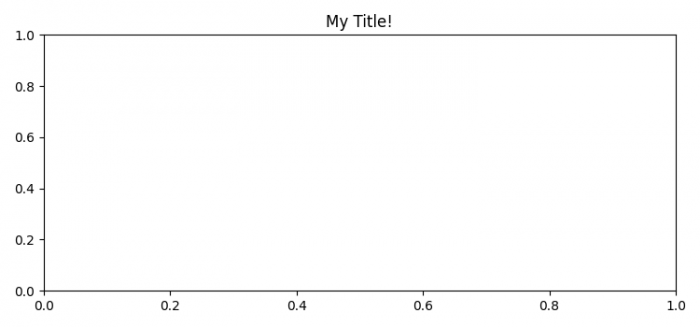# How can I attach a pyplot function to a figure instance? (Matplotlib)

To attach a pyplot function to a figure instance, we can use figure() method and add an axes to it.

## Steps

• Set the figure size and adjust the padding between and around the subplots.
• Create a new figure or activate an existing figure using figure() method.
• Add an '~.axes.Axes' to the figure as part of a subplot arrangement.
• Set a title to this axis using set_title() method.
• To display the figure, use show() method.

## Example

import matplotlib.pyplot as plt

plt.rcParams["figure.figsize"] = [7.50, 3.50]
plt.rcParams["figure.autolayout"] = True

fig = plt.figure()

plt.show()